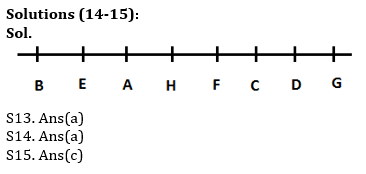Latest Banking jobs   »   Reasoning Ability Quiz For IBPS Clerk...

# Reasoning Ability Quiz For IBPS Clerk Prelims 2021- 23rd July

Directions (1-3): Study the following information carefully and answer the given questions.
Amongst five friends, A, B, C, D, E, each got different marks in the examination. A scored more than B but less than C. C scored 65 marks. D scored less marks than only E. The one who scored the minimum marks scored 60 marks and the one who scored the highest, scored 80 marks.

Q1. Who scored the second highest marks?
(a) B
(b) E
(c) D
(d) C
(e) A

Q2. Who is the most likely to have scored 62 marks?
(a) B
(b) A
(c) D
(d) E
(e) Either E or B

Q3. Who scored the lowest marks?
(a) B
(b) E
(c) D
(d) C
(e) A

Q4. In a class Nisha is 10th from the top and Aparna is 20th from the bottom. Naveen is 11 ranks above Aparna and 21 ranks below Nisha. How many students are there in the class?
(a) 60
(b) 61
(c) 62
(d) 58
(e) None of these

Q5. Rohit’s rank is 21st from the top and 15th from the bottom in the class. How many students are there in the class?
(a) 29
(b) 36
(c) 27
(d) 35
(e) None of these

Q6.How many pairs of letters are there in the word ‘CONFUSION’, each of which have as many letters between them in the word as they have between then in the English alphabet (both forward and backward direction)?
(a) Six
(b) Five
(c) Two
(d) Three
(e) One

Q7. Four of the following five are alike in certain way based from a group, find the one that does not belong to that group??
(a) EHL
(b) PSW
(c) ILP
(d) RUX
(e) GJN

Q8. In the given number ‘31756365842’ if ‘2’ is added to each odd number and ‘1’ is subtracted to each even number then how many digits are not repeated in the resultant thus obtained?
(a) one
(b) two
(c) three
(d) four
(e) None

Directions (9-12): Study the following information carefully and answer the given questions.
Amongst Six friends M, N, O, P, Q and R, each got different marks in the examination. P score just more than R. Not more than three persons score more marks than O. M score more than Q and less than R. P does not score maximum marks. The one who score least marks score 31 marks. Q score is not odd number. The one who score 4th highest score 52 marks.

Q9. Who scored the highest marks?
(a) N
(b) R
(c) Q
(d) M
(e) O

Q10. Who among the following Person has scored third highest marks?
(a) Q
(b) R
(c) N
(d) Q
(e) Either (b) or (c)

Q11. If P’s score 61 marks than what may be the score of R?
(a) 58
(b) 68
(c) 51
(d) 64
(e) None of these

Q12. Who among the following person score just more than M?
(a) O
(b) P
(c) N
(d) Q
(e) None of these

Q13. Kamal is 15th from the left end of a row of 45 students and Preet is 28th from the right end in the same row. How many students are there between them in the row?
(a) 3
(b) 2
(c) 4
(d) 1
(e) None of these

Directions (14-15): Study the following information carefully and answer the given questions.
Eight persons are sitting in a row facing south but not necessarily in the same order. H sits second to the right of C and second to the left of E. B is not an immediate neighbour of H and C. F is second to the right of D. G is not the neighbour of E. Only two persons sit to the right of A. G is not an immediate neighbour of A and doesn’t sit at extreme right end.

Q14. Who among the following is an immediate neighbour of both H and E?
(a) A
(b) B
(c) C
(d) G
(e) None of these

Q15. In which of the following pairs the second person is sitting on the right of the first person?
(a) A, H
(b) C, D
(c) G, H
(d) E, H
(e) F, D

Solutions

Solutions (1-3):
Sol. E(80) > D > C(65) > A > B (60)

S1. Ans.(c)
S2. Ans.(b)
S3. Ans.(a)

S4. Ans.(b)
Sol. Total number of students in the class = 61

S5. Ans.(d)
Sol. Total number of students in the class= (21+15)-1= 35

S6. Ans.(a)

S7.Ans(d)

S8.Ans.(b)
Sol. ‘31756365842’ changes to ‘53975557731’ two numbers i.e. 9 and 1 are not repeated.

Solutions (9-13):
Sol. O>P>R>M(52)>Q>N(31)

S9. Ans(e)
S10. Ans(b)
S11. Ans(a)
S12. Ans(e)

S13. Ans(b)
Sol. Kamal position from right end =(45+1-15)=31
Students between them=(31-28-1)=2